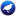# functions

This category contains 21 nodes.

##### GaussianProcesses (3.7)

Implements Gaussian processes for regression without hyperparameter-tuning

##### IsotonicRegression (3.7)

Learns an isotonic regression model

##### KernelLogisticRegression (3.7)

This classifier generates a two-class kernel logistic regression model

##### LibLINEAR (3.7)

A wrapper class for the liblinear classifier. Rong-En Fan, Kai-Wei Chang, Cho-Jui Hsieh, Xiang-Rui Wang, Chih-Jen Lin (2008)

##### LibSVM (3.7)

A wrapper class for the libsvm tools (the libsvm classes, typically the jar file, need to be in the classpath to use this classifier). LibSVM runs faster […]

##### LinearRegression (3.7)

Class for using linear regression for prediction

##### Logistic (3.7)

Class for building and using a multinomial logistic regression model with a ridge estimator. There are some modifications, however, compared to the paper of […]

##### MultilayerPerceptron (3.7)

A Classifier that uses backpropagation to classify instances. This network can be built by hand, created by an algorithm or both

##### PaceRegression (3.7)

Class for building pace regression linear models and using them for prediction

##### RBFClassifier (3.7)

Class implementing radial basis function networks for classification, trained in a fully supervised manner using WEKA's Optimization class by minimizing […]